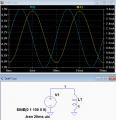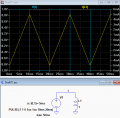# When does AC generate peak flux and current in a transformer?

Joined Oct 26, 2012
254
Does AC passing through an inductor generate more flux in the core during transitions? Or during steady (DC) state?
Does AC passing through an inductor generate more current in the secondary during transition? Or during steady (DC) state?

#### crutschow

Joined Mar 14, 2008
25,130
The maximum flux in a magnetic core occurs when the current is at a maximum, whether from a DC source or an AC source.
The maximum AC current in an inductor occurs when the AC voltage has returned to zero (for a sinewave excitation).
What "transitions" are you referring to?

Joined Oct 26, 2012
254
The maximum flux in a magnetic core occurs when the current is at a maximum
When is AC current at it's maximum?
The maximum AC current in an inductor occurs when the AC voltage has returned to zero (for a sinewave excitation).What "transitions" are you referring to?
I think that's the transition i'm referring to. The instant the V goes to zero from top of the waveform, that's the moment of fastest change, right? At the top of the wave (before the transition to 0), the V is changing very slowly. At 0, change is happening very quickly, right?

At the moment V changes direction, it's at that moment when there's greatest resistance, because current is still flowing in the contrary direction. Current must change direction at that moment, to obey the Voltage, right?

So, my question is, at the moment when current and voltage most oppose each other, that's the moment when the strongest flux is produced, and thus the strongest current in the secondary. Correct?

No, i think i have this wrong. I think you're saying AC current in primary is strongest when input current and input voltage most agree with each other (same direction)....?

...Last edited:

#### crutschow

Joined Mar 14, 2008
25,130
So, my question is, at the moment when current and voltage most oppose each other, that's the moment when the strongest flux is produced. Correct?
No.
In an inductor, voltage and current are out of phase, so peak current occurs at 90° phase from peak voltage.
As I said, the strongest flux is produced when the current is at a maximum.
It has nothing directly to do with voltage or "when current and voltage most oppose each other".

See the voltage and current simulation in an inductor below.
Not that peak current (and thus flux) occurs when the voltage is zero, and peak voltage occurs when the current is zero.Joined Oct 26, 2012
254
so, current and voltage in the primary are always exactly 90 degrees out of phase, regardless of number of turns, or core-type, or resistance, or frequency, or any other parameter?

this page says its <=90 deg. That seems to contradict your implication that the phase difference is always 90 deg. Is it 90 only with sines?

My understanding is that there's a direct relationship between current in the coil and EMF in the core. i'm asking about that relationship between current and EMF.

This formula seems to indicate that EMF increases as rate of change of current increases, regardless of the phase relationship between voltage and current.E(t) = -L dI/dt

Meaning, i think, when current change-slope is fastest, we'll get highest flux. Ie, when current crosses 0. Doesn't matter what the voltage is doing, that's not in the equation.

That seems to contradict your comment that current is highest when V crosses 0.

Thx

Last edited:

#### crutschow

Joined Mar 14, 2008
25,130
so, current and voltage in the primary are always exactly 90 degrees out of phase, regardless of number of turns, or core-type, or resistance, or frequency, or any other parameter?
That's true for the transformer magnetizing current.
The load current is in phase with the voltage.
It's 90° if there's no significant resistance in the circuit, which is generally true for a transformer's high inductance and low magnetizing current.
i think, when current change-slope is fastest, we'll get highest flux.
Think that all you like but it's not true.
You are still confusing flux with voltage.
To repeat for the umpteenth time, flux is proportional to current, not voltage.
The rate-of change of current (flux) it proportional to voltage.
E(t) = -L dI/dt

Meaning, i think, when current change-slope is fastest, we'll get highest flux
Nope.
That equation is stating that the voltage (not flux) across an inductor is proportional to the rate of change of the current with time.

Transposing the terms we get di/dt = -E(t) /L.
This means the the rate of current change in an inductor is proportional to the applied voltage divided by the inductance.
Thus the rate of current change is fastest at the peak of the sinewave voltage.

And the inductor current keeps increasing as long as there is any voltage applied (for half the sinewave), thus the peak inductor current doesn't occur until the voltage returns to zero.

Below is the simulation with an applied square-wave that may help in your understanding.
Since the square-wave applies a constant voltage, the current-change slope is also constant.Last edited:

Joined Oct 26, 2012
254
What does the circled value represent?
Um... EMF? Electromagnetic Force? measured in Volts?So... if Volts is a measure of difference between 2 points, then i guess the EMF of the coil means the potential difference between the two ends of the primary coil, measured in volts?

That's true for the transformer magnetizing current. The load current is in phase with the voltage.
i guess you mean the voltage in the primary? Is this correct?
• magnetizing current: current flowing in the primary, due to electrical connection. With sinewaves, will be 90 deg out of phase with voltage in the primary.
• load current: current flowing in the secondary, due to induction. Always in phase with voltage in the primary.
It's 90° if there's no significant resistance in the circuit, which is generally true for a transformer's high inductance and low magnetizing current.

i think, when current change-slope is fastest, we'll get highest flux.
Think that all you like but it's not true. You are still confusing flux with voltage.
Maybe, but i think I know the difference between Voltage (electrical pressure or force due to potential difference) and Flux (magnetism). I think my confusion is otherwise.

To repeat for the umpteenth time, flux is proportional to current, not voltage.
Actually, just 3 times. You can repeat it umpteenth times if you like, but repetition and impatience don't create understanding. No need to be a curmudgeonThat equation is stating that the voltage (not flux) across an inductor is proportional to the rate of change of the current with time.
ok.... so the faster the primary current is changing, the higher the voltage across the primary, right?

Below is the simulation with an applied square-wave that may help in your understanding.
Are both those traces on the primary side? It appears that, with a square wave, current peaks at the instant the square wave changes state from positive to negative, correct?

#### crutschow

Joined Mar 14, 2008
25,130
if Volts is a measure of difference between 2 points, then i guess the EMF of the coil means the potential difference between the two ends of the primary coil, measured in volts?
Yes.
i guess you mean the voltage in the primary? Is this correct?
Correct.
Maybe, but i think I know the difference between Voltage (electrical pressure or force due to potential difference) and Flux (magnetism).
Okay, but you repeatedly used the word "flux" when you should have used the word "voltage" so it seemed like you were confused about that.
You can repeat it umpteenth times if you like, but repetition and impatience don't create understanding. No need to be a curmudgeon
But I am.
Look at the bottom of my posts.so the faster the primary current is changing, the higher the voltage across the primary, right?
Yes.
It's actually the primary voltage that is causing the change in current, of course.
Are both those traces on the primary side? It appears that, with a square wave, current peaks at the instant the square wave changes state from positive to negative, correct?
That's the primary voltage and consequent primary magnetizing current.

And yes, the current peaks at the point the square-wave changes state, since the inductor current keeps increasing as long as the voltage is applied.

#### DickCappels

Joined Aug 21, 2008
6,462

Joined Oct 26, 2012
254
If you include the effects of a core,

View attachment 160923
Is that the the primary voltage and consequent primary magnetizing current?

Why doesn't comment #4 look like that?

Thx

#### DickCappels

Joined Aug 21, 2008
6,462
Almost. This was an experiment in which the secondary was treated as a low voltage primary.

The difference between the waveform in post #4 and the one in post #10 is that the simulated inductor in post #4 is ideal -no real world distortion, and for some cases it is a very good representation of real world waveforms.

The waveforms in post #10 were obtained when driving an inexpensive, unloaded transformer. If you look at the current waveform you can see the effects of hysteresis in the steel core. The sine wave is distorted because the winding has resistance and that along with the huge magnetizing current (all of the current in the waveform is magnetizing current since there is not load) results in distortion of the voltage waveform. If there was a load on the secondary the waveform would look more like a sine wave. Sorry I failed to mention that in the earlier post.

#### crutschow

Joined Mar 14, 2008
25,130
along with the huge magnetizing current
Normally magnetizing current is quite low as compared to the transformer load current rating.

•DickCappels